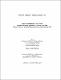## Lumping methods for model reduction2013
##### Authors
Okeke, Blessing Eberechukwu
University of Lethbridge. Faculty of Arts and Science
##### Publisher
Lethbridge, Alta. : University of Lethbridge, Dept. of Chemistry and Biochemistry, 2013
##### Abstract
Modelling a chemical or biochemical system involves the use of differential equations which often include both fast and slow time scales. After the decay of transients, the behaviour of these differential equations usually rests on a low-dimensional surface in the phase space which is called the slow invariant manifold (SIM) of the flow. A model has been effectively reduced if such a manifold can be obtained. In this study, we develop a method that introduces the lumping process (a technique whereby chemical species are grouped into pseudo-reagents (lumps) to simplify modelling) into the invariant equation method, and this new method effectively reduces complex models as well as preserving the structure and underlying mechanism of the original system. We also apply these methods to simple models of metabolic pathways. This method of model reduction would be of great importance for industrial applications
##### Description
ix, 93 leaves ; 29 cm
##### Keywords
Model reduction , Lumping process , Methods for model reduction , Dissertations, Academic , Biochemistry -- Mathematical models , Chemical reactions , Biochemistry